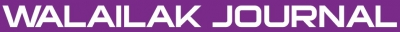A Comparison between Solving of Two-Dimensional Nonlinear Fredholm Integral Equations of the Second Kind by the Optimal Homotopy Asymptotic Method and Homotopy Perturbation Method

Abstract

In this article, we present the optimal homotopy asymptotic method (OHAM) and homotopy perturbation method (HPM) for solving 2-dimensional nonlinear Fredholm integral equations of the second kind. A comparison is made between these methods to solve 2-dimensional nonlinear Fredholm integral equations of the second kind. The results show that the presented methods are very powerful and simple techniques in solving 2-dimensional nonlinear Fredholm integral equations of the second kind.

doi:10.14456/WJST.2015.35

Keywords

Optimal homotopy asymptotic method, homotopy perturbation method, 2-dimensional nonlinear Fredholm integral equations of the second kind

PDF

References

M Abdou, L El-Kalla and A Al-Bugami. New approach for convergence of the series solution to a class of Hammerstein integral equations. Int. J. Appl. Math. Comput. 2011; 3, 261-9.

Z Avazzadeh and M Heydari. Chebyshev polynomials for solving two dimensional linear and nonlinear integral equations of the second kind. Comput. Appl. Math. 2012; 31, 127-42.

E Babolian, S Bazm and P Lima. Numerical solution of nonlinear two-dimensional integral equations using rationalized Haar functions. Comm. Nonlinear Sci. Numer. Simulat. 2011; 16, 1164-75.

H Guoqiang and W Jiong. Extrapolation of nystrom solution for two-dimensional nonlinear Fredholm integral equations. J. Comput. Appl. Math. 2001; 134, 259-68.

M Heydari, Z Avazzadeh, H Navabpour and G Loghmani. Numerical solution of Fredholm integral equations of the second kind by using integral mean value theorem II. High dimensional problems. Appl. Math. Model. 2013; 37, 432-42.

W Xie and F Lin. A fast numerical solution method for two dimensional Fredholm integral equations of the second kind. Appl. Math. Comput. 2009; 59, 1709-19.

J He. Homotopy perturbation technique. Comput. Meth. Appl. Mech. Eng. 1999; 178, 257-62.

J He. A coupling method of a homotopy technique and a perturbation technique for nonlinear problems. Int. J. Nonlinear Mech. 2000; 35, 37-43.

J He. The homotopy perturbation method for nonlinear oscillators with discontinuities. Appl. Math. Comput. 2004; 151, 287-92.

J He. Comparison of homotopy perturbation method and homotopy analysis method. Appl. Math. Comput. 2004; 156, 527-39.

J He. Some asymptotic methods for strongly nonlinear equations. Int. J. Mod. Phys. B 2006; 20, 1141-99.

J He. Homotopy perturbation method for solving boundary value problems. Phys. Lett. A 2006; 50, 87-8.

M El-Shahed. Application of He’s Homotopy perturbation method to Volterra’s integro-differential equation. Int. J. Nonlinear Sci. Numer. Simulat. 2005; 6,163-8.

AA Hemeda. Homotopy perturbation method for solving systems of nonlinear coupled equations. Appl. Math. Sci. 2012; 6, 4787-800.

S Aminsadrabad. Solution for inverse space-dependent heat source problems by homotopy perturbation method. Appl. Math. Sci. 2012; 6, 575 - 8.

N Herişanu, V Marinca, T Dordea and G Madescu. A new analytical approach to nonlinear vibration of an electrical machine. Proc. Rom. Acad. Ser. A 2008; 9, 229-36.

V Marinca and N Herisanu. Application of optimal homotopy asymptotic method for solving non-linear equations arising in heat transfer. Int. Comm. Heat Mass Tran. 2008; 35, 710-5.

V Marinca, N Herisanu and I Nemes. Optimal homotopy asymptotic method with application to thin film flow. Cent. Eur. J. Phys. 2008; 6, 648-53.

V Marinca, N Herisanu, C Bota and B Marinca. An optimal homotopy asymptotic method applied to the steady flow of a fourth-grade fluid past a porous plate. Appl. Math. Lett. 2009; 22, 245-51.

MS Hashmi, N Khan and S Iqbal. Numerical solutions of weakly singular Volterra integral equations using the optimal homotopy asymptotic method. Comput. Math. Appl. 2012; 64, 1567-74.

NR Anakira, AK Alomari and I Hashim. Optimal homotopy asymptotic method for solving delay differential equations. Math. Prob. Eng. 2013; 2013, 498902.

M Almousa and A Ismail. Optimal homotopy asymptotic method for solving the linear Fredholm integral equations of the first kind. Abstr. Appl. Anal. 2013; 2013, 278097.

F Mabood, WA Khan and A Ismail. Analytical solution for radiation effects on heat transfer in Blasius flow. Int. J. Mod. Eng. Sci. 2013; 2, 63-72.

F Mabood, WA Khan and A Ismail. Series solution for steady heat transfer in a heat-generating fin with convection and radiation. Math. Prob. Eng. 2013; 2013, 806873.

Refbacks

• There are currently no refbacks.Online ISSN: 2228-835X

http://wjst.wu.ac.th

Last updated: 17 May 2019